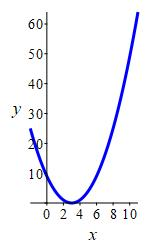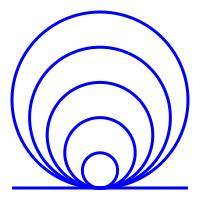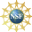# 8. Properties of Curves

## 1. Curvature

The curvature of a curve, denoted by the Greek letter kappa, $$\kappa$$, is a measure of how much a curve is bending. For example, the parabola at the right is more curved near $$x=2$$ than near $$x=8.$$Similarly, a circle with a small radius is more curved than a circle with a large radius, and a straight line (a circle with infinite radius) has no curvature at all. In fact, as we will see shortly, the curvature of a circle is the reciprocal of its radius: $\kappa=\dfrac{1}{R}$ We measure the curvature by calculating how fast the unit tangent vector is changing direction with respect to arc length.The curvature, $$\kappa$$, of a parametric curve, $$\vec{r}(t)$$, is $\kappa=\left|\dfrac{d\hat{T}}{ds}\right|$ where $$\hat{T}$$ is the unit tangent vector to the curve and $$s$$ is the arc length parameter on the curve.

1) We use the unit tangent vector $$\hat{T}$$ instead of the velocity vector $$\vec{v}$$ because $$\vec{v}$$ includes information about the speed $$|\vec{v}|$$ while the curvature is only supposed to measure the change in the direction of the tangent vector which is $$\hat{T}$$.

2) We take the derivative with respect to arc length $$s$$ instead of the parameter $$t$$ because we don't want the curvature to depand on how fast we travel on the curve. So $$\dfrac{d\hat{T}}{ds}$$ measures the rate of change of the direction of the tangent vector, $$\hat{T}$$, with respect to arc length, $$s$$.
3) We take the magnitude of $$\dfrac{d\hat{T}}{ds}$$ because we want the curvature to be a single number rather than a vector.

It is often easier to calculate curvature using one of the following formulas:

The curvature can be calculated from: $\kappa=\dfrac{1}{|\vec{v}|}\left|\dfrac{d\hat{T}}{dt}\right| =\dfrac{|\vec{v}\times\vec{a}|}{|\vec{v}|^{3}}$

First, using the Chain Rule and the formula for the speed $$\dfrac{ds}{dt}=|\vec{v}|$$ we have $\dfrac{d\hat{T}}{ds}=\dfrac{dt}{ds}\dfrac{d\hat{T}}{dt} =\dfrac{1}{|\vec{v}|}\dfrac{d\hat{T}}{dt}$ So $\kappa=\left|\dfrac{d\hat{T}}{ds}\right| =\dfrac{1}{|\vec{v}|}\left|\dfrac{d\hat{T}}{dt}\right|$ Next, using the Product Rule to differentiate the unit tangent vector $$\hat{T}=\dfrac{1}{|\vec{v}|}\vec{v}$$ we have $\dfrac{d\hat{T}}{dt} =\dfrac{1}{|\vec{v}|}\dfrac{d\vec{v}}{dt} +\dfrac{-1}{|\vec{v}|^2}\dfrac{d|\vec{v}|}{dt}\vec{v}$ Now $$\dfrac{d\vec{v}}{dt}=\vec{a}$$ and \begin{aligned} \dfrac{d|\vec{v}|}{dt} &=\dfrac{d}{dt}\sqrt{\vec{v}\cdot\vec{v}} =\dfrac{1}{2\sqrt{\vec{v}\cdot\vec{v}}} \left(\dfrac{d\vec{v}}{dt}\cdot\vec{v}+\vec{v}\cdot\dfrac{d\vec{v}}{dt}\right) \\ &=\dfrac{1}{2|\vec{v}|}\left(2\dfrac{d\vec{v}}{dt}\cdot\vec{v}\right) =\dfrac{\vec{a}\cdot\vec{v}}{|\vec{v}|} \end{aligned} So $\dfrac{d\hat{T}}{dt} =\dfrac{1}{|\vec{v}|}\vec{a} +\dfrac{-1}{|\vec{v}|^2}\dfrac{\vec{a}\cdot\vec{v}}{|\vec{v}|}\vec{v} =\dfrac{1}{|\vec{v}|} \left(\vec{a}-\,\dfrac{\vec{a}\cdot\vec{v}}{|\vec{v}|^2}\vec{v}\right)$ Now, $$\vec{a}-\,\dfrac{\vec{a}\cdot\vec{v}}{|\vec{v}|^2}\vec{v} =\text{proj}_{\bot \vec{v}}\vec{a}$$ and we saw on the previous page that $$\left|\text{proj}_{\bot \vec{v}}\vec{a}\right| =\dfrac{|\vec{v}\times\vec{a}|}{|\vec{v}|}$$. So: $\left|\dfrac{d\hat{T}}{dt}\right| =\dfrac{1}{|\vec{v}|}\left|\text{proj}_{\bot \vec{v}}\vec{a}\right| =\dfrac{|\vec{v}\times\vec{a}|}{|\vec{v}|^2}$ Therefore: $\kappa=\dfrac{1}{|\vec{v}|}\left|\dfrac{d\hat{T}}{dt}\right| =\dfrac{|\vec{v}\times\vec{a}|}{|\vec{v}|^{3}}$

Find the curvature $$\kappa$$ of the twisted cubic $$\vec{r}=\left\langle t,t^2,\dfrac{2}{3}t^{3}\right\rangle$$.

On the previous page, in the example to find $$\hat{T}$$, $$\hat{N}$$ and $$\hat{B}$$ for this twisted cubic, we found: \begin{aligned} &\vec{v}=\langle 1,2t,2t^2\rangle &&|\vec{v}|=1+2t^2 \\ &\vec{a}=\langle 0,2,4t\rangle &&|\vec{v}\times\vec{a}|=2(1+2t^2) \end{aligned} So $\kappa=\dfrac{|\vec{v}\times\vec{a}|}{|\vec{v}|^{3}} =\dfrac{2(1+2t^2)}{(1+2t^2)^{3}}=\dfrac{2}{(1+2t^2)^2}$

Find the curvature $$\kappa$$ of the circle $$\vec{r}(\theta)=\langle R\cos\theta,R\sin\theta,0\rangle$$.

$$\kappa=\dfrac{1}{R}$$

On a previous page, in the example to find $$\hat{T}$$, $$\hat{N}$$ and $$\hat{B}$$ for this circle, we found: \begin{aligned} &\vec{v}=\langle -R\sin\theta,R\cos\theta,0\rangle &&|\vec{v}|=R \\ &\vec{a}=\left\langle -R\cos\theta,-R\sin\theta,0\right\rangle &&|\vec{v}\times\vec{a}|=R^2 \end{aligned} So $\kappa=\dfrac{|\vec{v}\times\vec{a}|}{|\vec{v}|^{3}} =\dfrac{R^2}{R^{3}}=\dfrac{1}{R}$

As promised, the curvature, $$\kappa$$, of a circle is the reciprocal of its radius, $$R$$. Based on this fact, we define:

The radius of curvature of a curve is the reciprocal of its curvature: $R=\dfrac{1}{\kappa}$

Thus, the radius of curvature at a point on the curve is the radius of the circle which best fits the curve at that point. That circle is called the .

The word osculate comes from the Latin word osculum which means to kiss. So go home and ask your significant other if they would like to osculate tonight.

The osculating circle at a point on a curve is the circle which instantaneously lies in the same plane as the circle (the $$\hat T\hat N$$-plane), is tangent to the curve at that point, bends in the same direction as the curve and has radius equal to the radius of curvature of the curve at that point. It is regarded as the best fit circle at that point.

If $$R$$ is the radius of curvature at a point $$\vec{r}(a)$$ on a curve $$\vec{r}(t)$$, then the osculating circle at the point $$\vec{r}(a)$$ may be parametrized by: $\vec{r}_a(\phi) =\vec{r}(a)+R\vec{N}+R\cos\phi\,\hat{T}+R\sin\phi\,\hat{N}$

#### Derivation

We first find the center of the circle. Starting from the point $$\vec{r}(a)$$ on the curve, we move a distance $$R$$ in the direction perpendicular to the curve on the side the curve is bending, i.e. in the direction $$\hat N$$. So the center is at $$P=\vec{r}(a)+R\vec{N}$$. A circle centered at the origin in the $$\hat T\hat N$$-plane may be parametrized by $$\vec{r}(\phi)=R\cos\phi\,\hat{T}+R\sin\phi\,\hat{N}$$. Moving this so it is centered at $$P$$, gives the osculating circle parametrized by: $\vec{r}_a(\phi) =\vec{r}(a)+R\vec{N}+R\cos\phi\,\hat{T}+R\sin\phi\,\hat{N}$

Find the curvature $$\kappa$$ and radius of curvature $$R$$ of the helix $$\vec{r}(\theta)=\langle 4\cos\theta,4\sin\theta,3\theta\rangle$$.

$$\kappa=\dfrac{4}{25}$$   $$R=\dfrac{25}{4}$$

On a previous page, in the exercise to find $$\hat{T}$$, $$\hat{N}$$ and $$\hat{B}$$ for this helix, we found: \begin{aligned} &\vec{v}=\langle -4\sin\theta,4\cos\theta,3\rangle &&|\vec{v}|=5 \\ &\vec{a}=\langle -4\cos\theta,-4\sin\theta,0\rangle &&|\vec{v}\times\vec{a}|=20 \end{aligned} So the curvature is: $\kappa=\dfrac{|\vec{v}\times\vec{a}|}{|\vec{v}|^{3}} =\dfrac{20}{5^{3}}=\dfrac{4}{25}$ and then the radius of curvature is: $R=\dfrac{1}{\kappa}=\dfrac{25}{4}$

Find a parametrization for the osculating circle for this helix at the point $$\vec{r}(a)=\langle 4\cos a,4\sin a,3a\rangle$$.

The movie shows the osculating circle "rolling" along this helix.

Since the components are so long, we have written the vector as a column: $\vec{r}_a(\phi)= \begin{pmatrix} -\,\dfrac{9}{4}\cos a-5\sin a\cos\phi-\,\dfrac{25}{4}\cos a\sin\phi \\[8pt] -\,\dfrac{9}{4}\sin a+5\cos a\cos\phi-\,\dfrac{25}{4}\sin a\sin\phi \\[8pt] 3a+\dfrac{15}{4}\cos\phi \end{pmatrix}$

The osculating circle can be parameterized by $\vec{r}_a(\phi) =\vec{r}(a)+R\vec{N}+R\cos\phi\,\hat{T}+R\sin\phi\,\hat{N}$ We know the point is $$\vec{r}(a)=\langle 4\cos a,4\sin a,3 a\rangle$$ and the radius of curvature is $$R=\dfrac{1}{\kappa}=\dfrac{25}{4}$$. From the exercise on a previous page, we know: $\hat{T} =\left\langle -\,\dfrac{4}{5}\sin\theta,\dfrac{4}{5}\cos\theta,\dfrac{3}{5}\right\rangle \qquad \hat{N} =\langle -\cos\theta,-\sin\theta,0\rangle$ At the point where $$\theta=a$$ these become: $\hat{T} =\left\langle -\,\dfrac{4}{5}\sin a,\dfrac{4}{5}\cos a,\dfrac{3}{5}\right\rangle \qquad \hat{N} =\langle -\cos a,-\sin a,0\rangle$ Now we plug into the formula: (Since the components are so long, we switch to column vectors for the last two steps.) \begin{aligned} \vec{r}_a(\phi) &=\vec{r}(a)+R\vec{N}+R\cos\phi\,\hat{T}+R\sin\phi\,\hat{N} \\ &=\langle 4\cos a,4\sin a,3a\rangle +\dfrac{25}{4}\langle -\cos a,-\sin a,0\rangle \\ &+\dfrac{25}{4}\cos\phi\left\langle -\,\dfrac{4}{5}\sin a, \dfrac{4}{5}\cos a,\dfrac{3}{5}\right\rangle +\dfrac{25}{4}\sin\phi\langle -\cos a,-\sin a,0\rangle \\[8pt] &=\begin{pmatrix} 4\cos a-\,\dfrac{25}{4}\cos a-5\sin a\cos\phi-\,\dfrac{25}{4}\cos a\sin\phi \\[8pt] 4\sin a-\,\dfrac{25}{4}\sin a+5\cos a\cos\phi-\,\dfrac{25}{4}\sin a\sin\phi \\[8pt] 3a+\dfrac{15}{4}\cos\phi \end{pmatrix} \\[8pt] &=\begin{pmatrix} -\,\dfrac{9}{4}\cos a-5\sin a\cos\phi-\,\dfrac{25}{4}\cos a\sin\phi \\[8pt] -\,\dfrac{9}{4}\sin a+5\cos a\cos\phi-\,\dfrac{25}{4}\sin a\sin\phi \\[8pt] 3a+\dfrac{15}{4}\cos\phi \end{pmatrix} \end{aligned}

Supported in part by NSF Grant #1123255